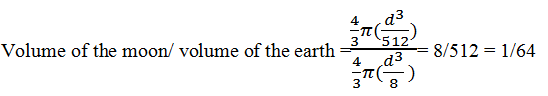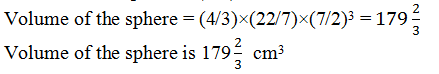# NCERT Solutions For Class 9 Math Chapter – 13 Exercise – 13.8

1. Find the volume of a sphere whose radius is

(i) 7 cm (ii) 0.63 m

(Assume π =22/7)

Solution:

(i) Radius of sphere, r = 7 cm

Using, Volume of sphere = (4/3) πr3

= (4/3)×(22/7)×73

= 4312/3

Hence, volume of the sphere is 4312/3 cm3

(ii) Radius of sphere, r = 0.63 m

Using, volume of sphere = (4/3) πr3

= (4/3)×(22/7)×0.633

= 1.0478

Hence, volume of the sphere is 1.05 m(approx).

2. Find the amount of water displaced by a solid spherical ball of diameter

(i) 28 cm (ii) 0.21 m

(Assume π =22/7)

Solution:

(i) Diameter = 28 cm

Radius, r = 28/2 cm = 14cm

Volume of the solid spherical ball = (4/3) πr3

Volume of the ball = (4/3)×(22/7)×14=

34496/3

Hence, volume of the ball is 34496/3 cm3

(ii) Diameter = 0.21 m

Radius of the ball =0.21/2 m= 0.105 m

Volume of the ball = (4/3 )πr3

Volume of the ball = (4/3)× (22/7)×0.1053 m3

Hence, volume of the ball = 0.004851 m3

3.The diameter of a metallic ball is 4.2cm. What is the mass of the ball, if the density of the metal is 8.9 g per cm3? (Assume π=22/7)

Solution:

Given,

Diameter of a metallic ball = 4.2 cm

Radius(r) of the metallic ball, r = 4.2/2 cm = 2.1 cm

Volume formula = 4/3 πr3

Volume of the metallic ball = (4/3)×(22/7)×2.1 cm3

Volume of the metallic ball = 38.808 cm3

Now, using relationship between, density, mass and volume,

Density = Mass/Volume

Mass = Density × volume

= (8.9×38.808) g

= 345.3912 g

Mass of the ball is 345.39 g (approx).

4. The diameter of the moon is approximately one-fourth of the diameter of the earth. What fraction of the volume of the earth is the volume of the moon?

Solution:

Let the diameter of earth be “d”. Therefore, the radius of earth will be will be d/2

Diameter of moon will be d/4 and the radius of moon will be d/8

Find the volume of the moon :

Volume of the moon = (4/3) πr= (4/3) π (d/8)3 = 4/3π(d3/512)

Find the volume of the earth :

Volume of the earth = (4/3) πr3= (4/3) π (d/2)3 = 4/3π(d3/8)

Fraction of the volume of the earth is the volume of the moonAnswer: Volume of moon is of the 1/64 volume of earth.

5. How many litres of milk can a hemispherical bowl of diameter 10.5cm hold? (Assume π = 22/7)

Solution:

Diameter of hemispherical bowl = 10.5 cm

Radius of hemispherical bowl, r = 10.5/2 cm = 5.25 cm

Formula for volume of the hemispherical bowl = (2/3) πr3

Volume of the hemispherical bowl = (2/3)×(22/7)×5.253 = 303.1875

Volume of the hemispherical bowl is 303.1875 cm3

Capacity of the bowl = (303.1875)/1000 L = 0.303 litres(approx.)

Therefore, hemispherical bowl can hold 0.303 litres of milk.

6. A hemi spherical tank is made up of an iron sheet 1cm thick. If the inner radius is 1 m, then find the volume of the iron used to make the tank. (Assume π = 22/7)

Solution:

Inner Radius of the tank, (r ) = 1m

Outer Radius (R ) = 1.01m

Volume of the iron used in the tank = (2/3) π(R3– r3)

Put values,

Volume of the iron used in the hemispherical tank = (2/3)×(22/7)×(1.013– 13) = 0.06348

So, volume of the iron used in the hemispherical tank is 0.06348 m3.

7. Find the volume of a sphere whose surface area is 154 cm2. (Assume π = 22/7)

Solution:

Let r be the radius of a sphere.

Surface area of sphere = 4πr2

4πr= 154 cm2 (given)

r2 = (154×7)/(4 ×22)

r = 7/2

Now,

Volume of the sphere = (4/3) πr38. A dome of a building is in the form of a hemi sphere. From inside, it was white-washed at the cost of Rs. 4989.60. If the cost of white-washing isRs20 per square meter, find the

(i) inside surface area of the dome (ii) volume of the air inside the dome

(Assume π = 22/7)

Solution:

(i) Cost of white-washing the dome from inside = Rs 4989.60

Cost of white-washing 1m2 area = Rs 20

CSA of the inner side of dome = 498.96/2 m2  = 249.48 m2

(ii) Let the inner radius of the hemispherical dome be r.

CSA of inner side of dome = 249.48 m2 (from (i))

Formula to find CSA of a hemi sphere = 2πr2

2πr2 = 249.48

2×(22/7)×r= 249.48

r= (249.48×7)/(2×22)

r= 39.69

r = 6.3

Volume of air inside the dome = Volume of hemispherical dome

Using formula, volume of the hemisphere = 2/3 πr3

= (2/3)×(22/7)×6.3×6.3×6.3

= 523.908

= 523.9(approx.)

Answer: Volume of air inside the dome is 523.9 m3.

9. Twenty-seven solid iron spheres, each of radius r and surface area S are melted to form a sphere with surface area S’. Find the

(i) radius r’ of the new sphere,

(ii) ratio of Sand S’.

Solution:

Volume of the solid sphere = (4/3)πr3

Volume of twenty seven solid sphere = 27×(4/3)πr3 = 36 π r3

(i) New solid iron sphere radius = r’

Volume of this new sphere = (4/3)π(r’)3

(4/3)π(r’)= 36 π r3

(r’)= 27r3

r’= 3r

Radius of new sphere will be 3r (thrice the radius of original sphere)

(ii) Surface area of iron sphere of radius r, S =4πr2

Surface area of iron sphere of radius r’= 4π (r’)2

Now

S/S’ = (4πr2)/( 4π (r’)2)

S/S’ = r2/(3r’)2 = 1/9

The ratio of S and S’ is 1: 9.

10. A capsule of medicine is in the shape of a sphere of diameter 3.5mm. How much medicine (in mm3) is needed to fill this capsule? (Assume π = 22/7)

Solution:

Diameter of capsule = 3.5 mm

Radius of capsule, say r = diameter/ 2 = (3.5/2) mm = 1.75mm

Volume of spherical capsule = 4/3 πr3

Volume of spherical capsule = (4/3)×(22/7)×(1.75)3 = 22.458

Answer: The volume of the spherical capsule is 22.46 mm3.